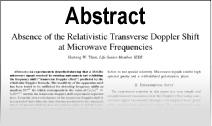Enter the content which will be displayed in sticky bar

AbstractAether, the Mother of All Forces in Nature - Nuclear Forces (Paper III of IV)

Year: 2018 Pages: 7
Keywords: Aether ocean, fluid, intrinsic pressure, strong force, weak force, binding energy, photon, negoff, posoff
Gravitational force and the nuclear strong force are established based on the same nature of the Aether fluid. However, because of the scales of distance involved in the establishment of these two forces are so incredibly different, some details that can be extremely trivial in the gravitational force becomes gravely important in bringing up the nuclear strong force. Upon recovering those "extremely trivial" details in the exploration of the strong force, we should find that the Aether's intrinsic pressure should be necessarily revised with a much higher magnitude, in the order of $\times 10^{14}Newton / cm^2$. This difference thus further brings in the contrasting difference in magnitude between these two forces. Since both of the forces are actually of the same nature, we categorizes them with one new term: condensive force. Although the nuclear strong force never exists without the presence of the Aether's intrinsic pressure, it is a short range force, acting in distance in the order of a nucleus. In such range, the Aether fluid pressure is escalated many folds above its normal intrinsic pressure. This creates a situation in which the strong force also competes with the Aether's intrinsic pressure. Their competition results in the so called weak force. If the acceptance of Aether would lead to a reasonable explanation of the condensive force and electromagnetic force, coupled with the finding that the theory of relativity is mathematically self-refuted $$_{}$$, the science world may find itself difficult to resign from the task to re-evaluate the validity of the Quantum Theory. At least, as the article develops, the concept of photon is found to be self-refuted, and so is Bohr's model regarding atomic structure.